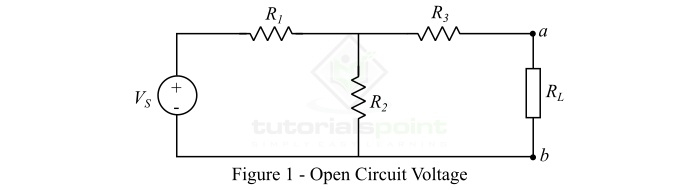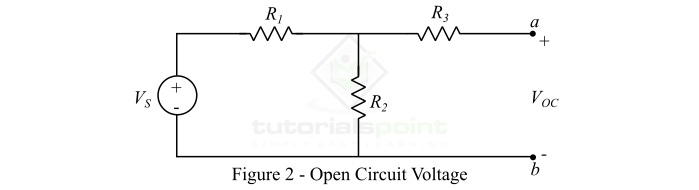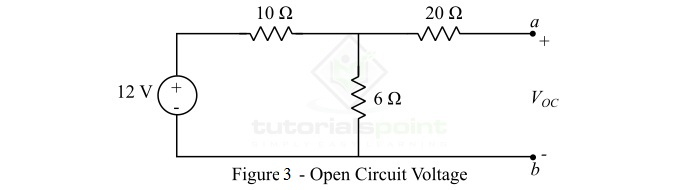# What is Open Circuit Voltage? – Definition, Calculation, and Example

In electrical and electronic circuits, the difference between potentials of two points is referred to as voltage or potential difference. Sometime we come across a term "open circuit voltage" while analyzing electric circuits. The term open circuit voltage is very common when we use TheveninтАЩs Theorem to analyze the circuit. Therefore, in this article, we will discuss what the open circuit voltage is, and how to calculate and test it. So letтАЩs begin with a basic introduction of the open circuit voltage.

## What is Open Circuit Voltage?

In an electric circuit, when a gap or open is created, and the difference of potentials between the two points of the open is referred to as the open circuit voltage. In circuit analysis terminology, we usually refer to the open circuit voltage as the TheveninтАЩs Voltage because the calculation of the open circuit voltage is used in TheveninтАЩs Theorem. The open circuit voltage is often represented by the symbol ЁЭСЙOC. The open circuit voltage is commonly used to express the voltage or potential difference of solar cells and batteries.

## Determination of Open Circuit Voltage

In an electric circuit, when we need to determine the open circuit voltage, the entire load of the circuit is removed. Under this condition, the only voltage drop occurs in the internal resistance of the source like battery or solar cell. However, this internal voltage drop is very small. Now, we simply use a voltmeter or a multimeter to measure the voltage between the open circuited terminals.

In circuit analysis, we use a standard procedure for the determination of the open circuit voltage, which is explained below. Consider an electric circuit a shown in Figure-1.Here, we have to determine the open circuited voltage across the load terminals ab. We do this in the following steps тИТ

Step 1 тИТ We will remove the load ЁЭСЕЁЭР┐ from the circuit, and mark the voltage between open terminals as ЁЭСЙOC as shown in Figure-2.Step 2 тИТ By inspection, it is clear that there is no current flowing through the resistance ЁЭСЕ3, hence there is no voltage drop across ЁЭСЕ3. Consequently, the open circuit voltage is equal to the voltage drop across the resistance ЁЭСЕ2.

Step 3 тИТ Using simple voltage division rule, the voltage across the resistance ЁЭСЕ2 is given by,

$$\mathrm{V_{R2}=\frac{V_{S}R_{2}}{R_{1}+R_{2}}}$$

Step 4 тИТ Since the voltage across the resistor ЁЭСЕ2 and the open circuit voltage are equal, i.e.,

$$\mathrm{V_{OC}=V_{R2}=\frac{V_{S}R_{2}}{R_{1}+R_{2}}}$$

In this way, we often determine the open circuit voltage in an electric circuit.

## What is Open Circuit Voltage Test?

The open circuit voltage test is mainly used to determine the electric potential capability of a battery and solar cells. This test gives the value of voltage or potential difference between the positive and negative terminals of the battery. We can use the open circuit voltage test for both primary and secondary (rechargeable) batteries. The data collected in the open circuit voltage test is used to calculate the state of charge (SOC) for a rechargeable battery.

When we refer the datasheet of a battery, the voltage mentioned on the battery is its open circuit voltage defined by the manufacturer. The open circuit voltage test on a battery is performed using a multimeter. For this, we set the multimeter knob on dc voltage, and measure the voltage across terminals of the battery.

For primary batteries, the open circuit voltage test is performed to check the state of the battery, i.e. the battery is damaged or not. While for rechargeable batteries, the open circuit voltage test is used to check whether the battery is charged or discharged.

## Why the Open Circuit Voltage is not Zero?

As we know, the voltage is the difference of potential between two points. This means, the two points are not connected together, or we can say these two points are connected to two different electric potentials.

In case of an open circuit, the two points are not connected to each other, but each of these two points is connected to the terminals of a voltage source like battery. Since both terminals of a battery have different electric potential levels, and hence the two points of the open circuit. In this way, a potential different is created between the two points of the open circuit, as a result, the open circuit voltage is not zero.

Note тИТ In case of a solar cell, the maximum voltage is available at the condition of zero current. Thus, the maximum voltage of solar cell is its open-circuit voltage.

## Numerical Example

Determine the open circuit voltage across the load terminals ab of the circuit shown in Figure-3.### Solution

In the given circuit, the open circuit voltage is equal to the voltage across 6╬й resistor as there is no current flowing through the 20╬й resistor. Hence, using voltage division rule, we get,

$$\mathrm{V_{OC}=V_{6\Omega }=\frac{12\times 6}{10+6}}$$

$$\mathrm{V_{OC}=4.5\, V}$$